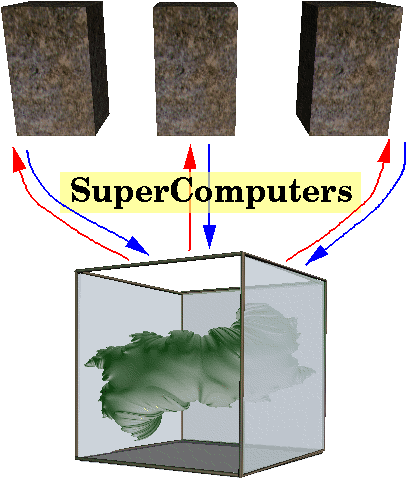This page is a documentation of the Quaternion Julia Sets in Virtual Reality project for SUPERCOMPUTING '95. I worked on this project in collaboration with Daniel J. Sandin, also of EVL, and Louis H. Kauffman and Yumei Dang, of the Department of Mathematics, Statistics, and Computer Science at the University of Illinois at Chicago.

## what they are

A Julia Set is the map of the behavior of an equation when its output is fed back into its input (recursive). The equation mapped is z=z**2+k. In general, the most common Julia Set is a map of that equation in complex numbers (2D numbers). In this case the equation is being mapped in quaternions, which are 4D hyper-complex numbers. This project investigates a method for visualizing Julia Sets based on estimating the distance from the viewpoint to the surface of the Julia Set.

Here are some example images:(larger version)

for more images, check out Yumei's page.

## in the CAVE

Because the process of rendering these forms is so computationally heavy, there was a need to use as much computational power as was possible. My primary contribution to this project was to supply this power. To do this I used the CAVEcomm library developed at the Mathematics and Computer Science Division of Argonne National Laboratory to communicate between the onyx that runs the CAVE and three seperate supercomputers that did all the calculations.Even with three supercomputers working on ray tracing the Julia Set, displaying it in real time is impossible. In order to eleviate the problem of having nothing to look at while all this computation was going on, we successively refined the image. This meant first ray tracing the set in low resolution and displaying it on the walls of the CAVE. We could then use the information from the low resolution image to determine which areas had the most contrast. Those areas could then be refined first, as they tend to be more interesting.

It was my responsability to establish the connections between the CAVE and the supercomputers, and keep track of all the information that was being sent back and forth. I received the pertinent data that would be needed to do the calculations from the CAVE. That data was then sent to which ever of the three supercomputers was least busy. The supercomputer would calculate the appropriate section of the image based on the information it received, and send the result back. I took those results and gave them back to the CAVE, which displayed it in the appropriate position.

back to NOW

back to insley on the internet